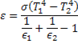# Previous Years GATE Questions on Radiation

## Solved GATE Questions on Radiation

### Question 1. For the circular tube of equal length and diameter shown below, the view factor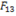is 0.17. The view factor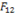in this case will be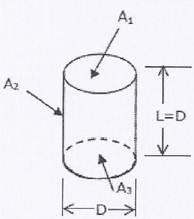(A) 0.17

(B) 0.21

(C) 0.79

(D) 0.83

GATE-ME-2001

Hint 1. (Ans D)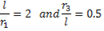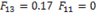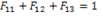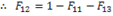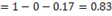(A) 0.83

(B) 1.17

(C) 0.66

(D) 1.34

GATE-ME-2002

Hint 2. (Ans A)

### Question 3. A plate having 10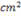area each side is hanging in the middle of a room of 100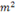total surface area. The plate temperature and emissivity are respectively 800 K and 0.6. The temperature and emissivity values for the surfaces of the room are 300 K and 0.3 respectively. Boltzmann’s constant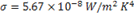. The total heat loss from the two surfaces of the plate is

(A) 13.66 W

(B) 27.32 W

(C) 27.87 W

(D) 13.66 MW

GATE-ME-2003

Hint 3. (Ans B)

Heat loss from one surface of the plate,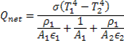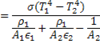Putting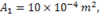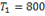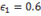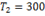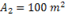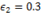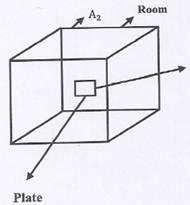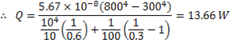Heat loss from both the surfaces =2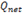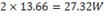### Question 4. A solar collector receiving solar radiation at the rate of 0.6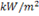transforms it to the internal energy of a fluid at an overall efficiency of 50 %. The fluid heated to 350 K is used to run a heat engine which rejects heat at 313 K. if the heat engine is to deliver 2.5 kW power, then minimum area of the solar collector required would be

(A) 8.33(B) 16.66(C) 39.68(D) 79.36GATE-ME-2004

Hint 1. (Ans B)

### The radiation configuration factor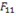is

(A) 0.375

(B) 0.625

(C) 0.75

(D) 1

GATE-ME-2005

Hint 5. (Ans C)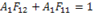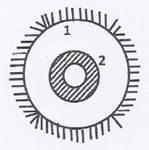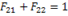Since,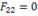, therefore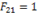Now,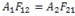Now,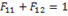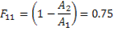### Question 6. The following figure was generated from experimental data relating spectral black body emissive power to wave length at three temperatures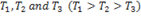.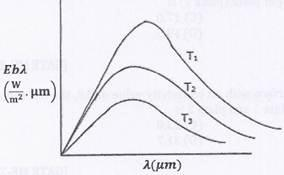### The conclusion is that the measurements are

(A) Correct because the maxima in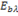show the correct trend.

(B) Correct because Planck’s law is satisfied.

(C) Wrong because the Stefan Boltzman law is not satisfied.

(D) Wrong because Wien’s displacement law is not satisfied

GATE-ME-2005

Hint 6. (Ans D)

When displacement law is not satisfied, i.e.,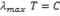Which tells lower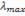for higher temperatures.

### Question 7. A hollow enclosure is formed between two infinitely long concentric cylinders of radii 1m and 2m, respectively. Radiative heat exchange takes place between the inner surface of the larger cylinder (surface-2) and the outer surface of the smaller cylinder (surface-1). The radiating surfaces are diffuse and the medium in the enclosure is non-participating. The fraction of the thermal radiation leaving the larger surface and striking itself is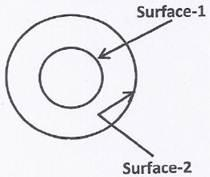(A) 0.25

(B) 0.5

(C) 0.75

(D) 1

GATE-ME-2008

Hint 7. (Ans B)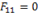due to concave surface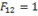Now,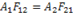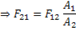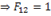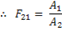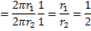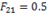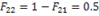Common data  Question 8 and 9

Radiative  heat transfer is intended between the inner surfaces of two very small isothermal parallel metal plates. While the upper plate (designated as plate 1) is a black surface and the warmer on being maintained at 727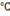, the lower plate (plate 2) is a diffuse and gray surface with an emissivity of 0.7 and is kept at 227. Assume that the surface are sufficiently large to form surface enclosure and steady state conditions to exist. Stefan Boltzmann constant is given as 5.67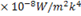.

### Question 8. The irradiation () for the upper plate (plate 1) is

(A) 2.5

(B) 3.6

(C) 17.0

(D) 19.5

GATE-ME-2009

Hint 8. (Ans A)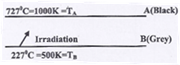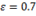Total irradiation for plate A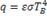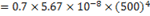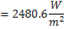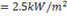### Question 9. If plate 1 is also a diffuse and gray surface with an emissivity value of 0.8, the net radiation heat exchange () between plate 1 and plate 2 is

(A) 17.0

(B) 19.5

(C) 23.0

(D) 31.7

GATE-ME-2009

Hint 9. (Ans D)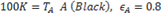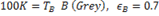Net heat exchange between A and B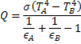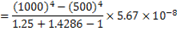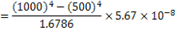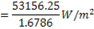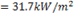### Question 10. For an opaque surface, the absorptivity () transmissivity () and reflectivity () are related by the equation:

(A)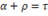(B)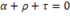(C)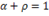(D)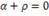GATE-ME-2012

Hint 10. (Ans C)

### Question 11. Two large diffuse gray parallel plates separated by a small distance, have surface temperature of 400 K and 300 K. If the emissivities of the surfaces are 0.8 and the Stefan-Boltzmann constant is 5.67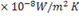, the net radiation heat exchange rate inbetween the two plates is

(A) 0.66

(B) 0.79

(C) 0.99

(D) 3.96

GATE-ME-2013

Hint 11. (Ans A)